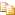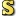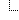﻿Rope(T) ClassAvalonEdit
Rope<(Of <(<'T>)>)> Class
NamespacesICSharpCode.AvalonEdit.UtilsRope<(Of <(<'T>)>)>
A kind of List<T>, but more efficient for random insertions/removal. Also has cheap Clone() and SubRope() implementations.Declaration Syntax
 C# Visual Basic Visual C++
```[SerializableAttribute]
public sealed class Rope<T> : IList<T>,
ICollection<T>, IEnumerable<T>, IEnumerable, ICloneable
```
```<SerializableAttribute> _
Public NotInheritable Class Rope(Of T) _
Implements IList(Of T), ICollection(Of T),  _
IEnumerable(Of T), IEnumerable, ICloneable```
```[SerializableAttribute]
generic<typename T>
public ref class Rope sealed : IList<T>,
ICollection<T>, IEnumerable<T>, IEnumerable, ICloneable```Generic Template Parameters
TMembers
 All Members Constructors Methods Properties PublicProtected InstanceStatic DeclaredInherited
IconMemberDescriptionRope<(Of <(<'T>)>)>()()()()
Creates a new rope representing the empty string.Rope<(Of <(<'T>)>)>(IEnumerable<(Of <<'(T>)>>))
Creates a rope from the specified input. This operation runs in O(N).Rope<(Of <(<'T>)>)>(array<T>[]()[][], Int32, Int32)
Creates a rope from a part of the array. This operation runs in O(N).Rope<(Of <(<'T>)>)>(Int32, Func<(Of <<'(Rope<(Of <<'(T>)>>)>)>>))
Creates a new rope that lazily initalizes its content.Add(T)
Appends the item at the end of the rope. Runs in O(lg N).AddRange(IEnumerable<(Of <<'(T>)>>))
Appends multiple elements to the end of this rope. Runs in O(lg N + M), where N is the length of this rope and M is the number of new elements.AddRange(Rope<(Of <<'(T>)>>))
Appends another rope to the end of this rope. Runs in O(lg N + lg M), plus a per-node cost as if newElements.Clone() was called.AddRange(array<T>[]()[][], Int32, Int32)
Appends new elements to the end of this rope. Runs in O(lg N + M), where N is the length of this rope and M is the number of new elements.Clear()()()()
Resets the rope to an empty list. Runs in O(1).Clone()()()()
Clones the rope. This operation runs in linear time to the number of rope nodes touched since the last clone was created. If you count the per-node cost to the operation modifying the rope (doing this doesn't increase the complexity of the modification operations); the remainder of Clone() runs in O(1).Concat(Rope<(Of <<'(T>)>>), Rope<(Of <<'(T>)>>))
Concatenates two ropes. The input ropes are not modified. Runs in O(lg N + lg M).Concat(array<Rope<(Of <<'(T>)>>)>[]()[][])
Concatenates multiple ropes. The input ropes are not modified.Contains(T)
Searches the item in the rope. Runs in O(N).CopyTo(array<T>[]()[][], Int32)
Copies the whole content of the rope into the specified array. Runs in O(N).CopyTo(Int32, array<T>[]()[][], Int32, Int32)
Copies the a part of the rope into the specified array. Runs in O(lg N + M).Count
Gets the length of the rope. Runs in O(1).Equals(Object)
Determines whether the specified Object is equal to the current Object.
(Inherited from Object.)GetEnumerator()()()()
Retrieves an enumerator to iterate through the rope. The enumerator will reflect the state of the rope from the GetEnumerator() call, further modifications to the rope will not be visible to the enumerator.GetHashCode()()()()
Serves as a hash function for a particular type.
(Inherited from Object.)GetRange(Int32, Int32)
Creates a new rope and initializes it with a part of this rope. Runs in O(lg N) plus a per-node cost as if this.Clone() was called.GetType()()()()
Gets the Type of the current instance.
(Inherited from Object.)IndexOf(T)
Finds the first occurance of item. Runs in O(N).IndexOf(T, Int32, Int32)
Gets the index of the first occurrence the specified item.Insert(Int32, T)
Inserts the item at the specified index in the rope. Runs in O(lg N).InsertRange(Int32, Rope<(Of <<'(T>)>>))
Inserts another rope into this rope. Runs in O(lg N + lg M), plus a per-node cost as if newElements.Clone() was called.InsertRange(Int32, IEnumerable<(Of <<'(T>)>>))
Inserts new elemetns into this rope. Runs in O(lg N + M), where N is the length of this rope and M is the number of new elements.InsertRange(Int32, array<T>[]()[][], Int32, Int32)
Inserts new elements into this rope. Runs in O(lg N + M), where N is the length of this rope and M is the number of new elements.Item[([( Int32])])
Gets/Sets a single character. Runs in O(lg N) for random access. Sequential read-only access benefits from a special optimization and runs in amortized O(1).LastIndexOf(T)
Gets the index of the last occurrence of the specified item in this rope.LastIndexOf(T, Int32, Int32)
Gets the index of the last occurrence of the specified item in this rope.Length
Gets the length of the rope. Runs in O(1).Remove(T)
Removes the first occurance of an item from the rope. Runs in O(N).RemoveAt(Int32)
Removes a single item from the rope. Runs in O(lg N).RemoveRange(Int32, Int32)
Removes a range of elements from the rope. Runs in O(lg N).SetRange(Int32, array<T>[]()[][], Int32, Int32)
Copies a range of the specified array into the rope, overwriting existing elements. Runs in O(lg N + M).ToArray()()()()
Creates an array and copies the contents of the rope into it. Runs in O(N).ToArray(Int32, Int32)
Creates an array and copies the contents of the rope into it. Runs in O(N).ToString()()()()
Creates a string from the rope. Runs in O(N).
(Overrides Object.ToString()()()().)Remarks
This class is not thread-safe: multiple concurrent write operations or writes concurrent to reads have undefined behaviour. Concurrent reads, however, are safe. However, clones of a rope are safe to use on other threads even though they share data with the original rope.Inheritance Hierarchy
 ObjectRope<(Of <(<'T>)>)>

Assembly: ICSharpCode.AvalonEdit (Module: ICSharpCode.AvalonEdit.dll) Version: 5.0.0.4238